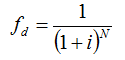# HOMER Grid 1.4The discount factor is a ratio used to calculate the present value of a cash flow that occurs in any year of the project lifetime. HOMER calculates the discount factor using the following equation:where: i N = number of years

Example: For i = 5% and N = 12 years, the discount factor equals 0.557. That means a \$1000 nominal cash flow in year 12 has a present value of \$557. In other words, a \$1000 cash flow in year 12 is equivalent to a \$557 cash flow in year zero. This is a demonstration of the time value of money; a dollar is now worth more than a dollar twelve years in the future.

The Homer Support site has a searchable knowledgebase and additional support options. HOMER Online contains the latest information on model updates, as well as sample files, resource data, and contact information. ©2017 HOMER Energy, LLC